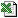ZeroCoupon (CalculationDate, ParBaseDate, ParDates, ParYields, ParCoupFreqs, CurrentPrices, [RateType], [DCount], [BusDaySwitch], [Options])
Determines the Zero Coupon Discount factor using the Bootstrap method
The function returns the either the Zero Coupon spot rate or the Zero Coupon Discount Factor.
Auto Array Function
###### Values for "Options" (or "Opts")

1 - Always Calculate Whole Curve. This is a performance optimization that you need to understand in order to use. The default behaviour of the function is only to use sufficient par dates and rates in order to construct the curve as far as the calculation date. Whilst this is efficient for making a few function calls at calculation dates near the start of the par rates, it does mean that the necessary part of the curve is calculated every time. If, however, you are making several function calls along the full length of the par rate curve, its best to calculate the whole curve the first time the function is called, and then get the function to use its internal cache of calculated rates for subsequent calls. Thats what this option does. Note that to get the very best performance, you should use this function as an array function (which always calculates the whole curve).

In other words: It does not affect the calculations, just the speed of processing. Option 0 is optimal for one or two calls to ZeroCoupon, because it only calculates the part of the curve that is needed. Option 1 says, OK, we are going to use the curve alot over many calls, so lets calculate the whole curve, and, provided we get the same set of inputs, we will not calculate the curve again, but use the curve we have stored internally. On the whole, Option 1 is what most people would want.

###### Key Points

Performance
The function has to create a zero coupon curve internally using the bootstrap method. If you are using this function for a range of Calculation Dates, eg converting a swap curve to a zero cpoupon curve, it will be much faster to use this function as an array function. To do this, you simply need to input a range of CalculationDates and use the array method of entering a formula.

In summary, your performance alternatives are:

• Do nothing: The function will calculate the curve as much as it needs to. In an example we carried out over 360 rates this resulted in an execution time of 32 seconds.
• Use the Options variable to instruct CalculateWholeCurve. The function will calculate the whole curve upon the first call and use the cahced calculations thereafter. In our example execution time was reduced to 2 seconds.
• Use as an array function. Specifying CalculateWholeCurve is superfluous since it will do this anyway. In our example execution time was reduced to 1 second.

###### Key Points

The Discount Factor is the factor by which you multiply a future value at CalculationDate to bring it back to ParBaseDate. This is quite a powerful function since it is calculating a bootstrapped zero coupon curve. For a less accurate way of working with spot and future rates see SpotToSpot and FutureToSpot.

###### ExampleZeroCoupon.xls

Business Functions Ltd, London, UK Website Design: Webpure